Vectors and conics

This free course is available to start right now. Review the full course description and key learning outcomes and create an account and enrol if you want a free statement of participation.

Free course

4.8 General equation of a conic

You have already met the parabola, ellipse and hyperbola. So far, you have considered the equation of a conic only when it is in standard form; that is, when the centre of the conic (if it has a centre) is at the origin, and the axes of the conic are parallel to the x- and y-axes. However, most of the conics that arise in calculations are not in standard form.

We have seen that any circle can be described by an equation of the form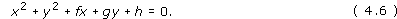More generally, it can be shown that any conic can be described by an equation of the form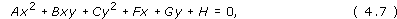where A, B and C are not all zero.

Equation (4.6) is of the form (4.7) with A = C = 1 and B = 0.

We know that the equations of the non-degenerate conics in standard form are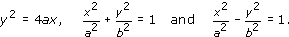Each of these equations is of the form (4.7).

The equations of degenerate conics can also be expressed in the form (4.7). For example: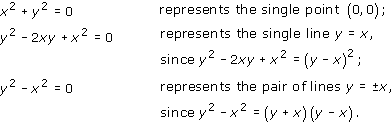However, an equation of the form (4.7) can also describe the empty set; an example of this is the equation x2 + y2 + 1 = 0, as there is no point (x, y) in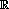2 for which x2 + y2 = −1. (This is an unexpected possibility!) For simplicity in the statement of the theorem below, therefore, we add the empty set to our existing list of degenerate conics.

Theorem 4

Any conic has an equation of the formwhere A, B, C, F, G and H are real numbers, and A, B and C are not all zero. Conversely, the set of all points in2 whose coordinates (x, y) satisfy an equation of the form (4.7) is a conic.

Note: We omit a proof of this result.

M208_1